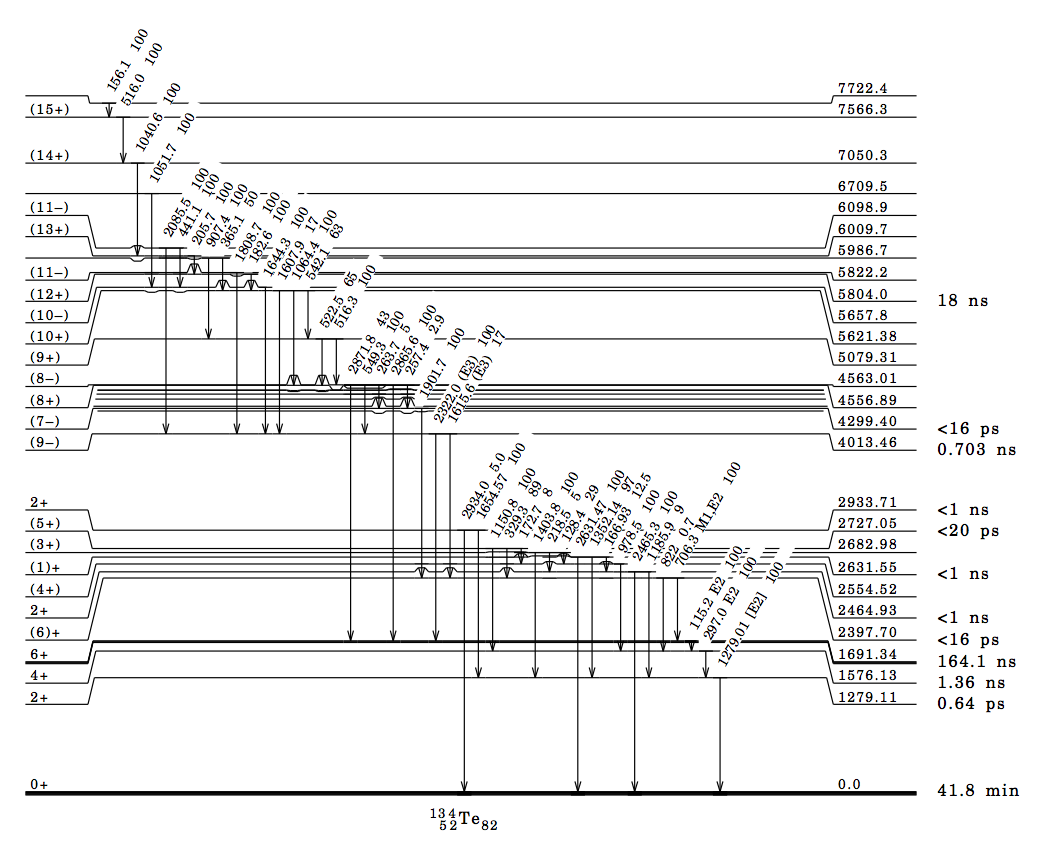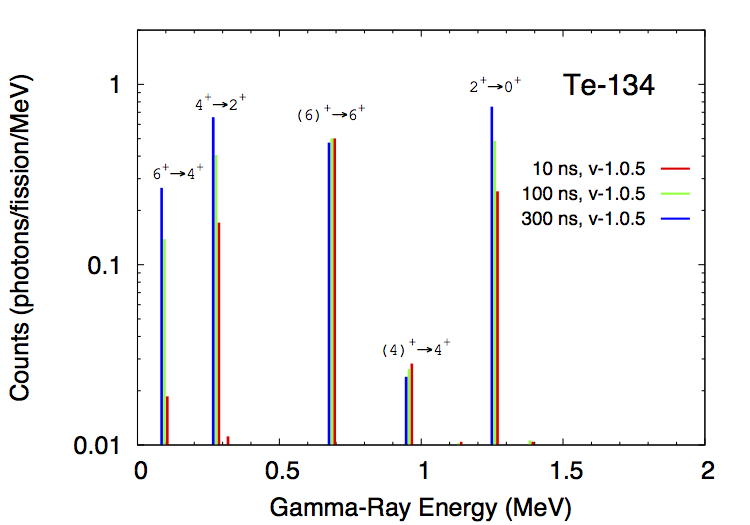# Isomeric States¶

Many low-lying discrete levels that are reported in the ENSDF database have a measurable half-life, ranging from nanoseconds to seconds and even longer. CGMF takes this into account when calculating the gamma cascades in the fission products, and samples the exponential decay law according to the reported half-lives.

An experimental time coincidence window can be set in the config.h configuration file:

const double EXPERIMENTAL_TIME_WINDOW = 1e-8;


The time is given in seconds, so in the example above, 1e-8 corresponds to 10 ns. The default value is negative. In this case, all levels are set to decay to the ground-state, ignoring half-lives entirely. Since this value is stored in a configuration file, it is set at compilation time. If the user decides to change this value, he/she would need to recompile the code before using it.

As an example, the calculated intensities for specific gamma lines in Te-134, in the thermal neutron-induced fission of U-235, are shown in the figure below. Time-coincidence windows of 10, 100 and 300 ns were used in three separate calculations. Because of the presence of ~100 ns isomers in Te-134, some of these lines are more or less prominent depending on their half-lives. For example, the $$6^+$$ state at 1.691 MeV has a half-life of 164 ns, decaying to the $$4^+$$ state at 1.576 MeV. A too-short time gate (e.g., 10ns) cannot record this particular gamma line at 115 keV. Similarly, the decay of the $$4^+$$ to $$2^+$$ (297 keV) is also hindered since it depends on the decay of the higher excited $$6^+$$ state.